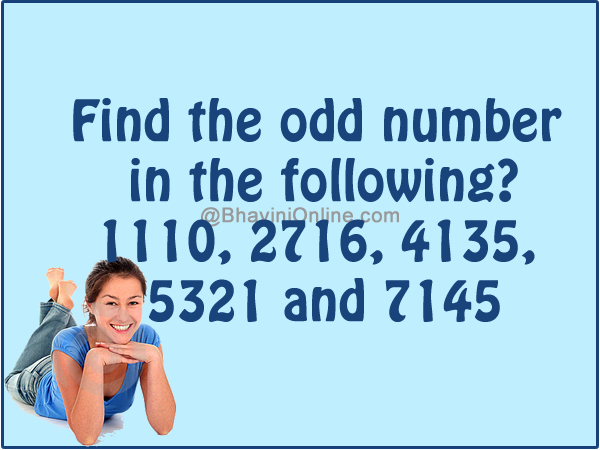# Find The Odd Number: 1110, 2716, 4135, 5321 And 7145

Have fun with numbers.

Find the odd number in the following?

1110, 2716, 4135, 5321 and 7145Share it when you get it.

If you go through the sequence you will see that the numbers add up to be a prime number.

Like;

1 + 1 + 1 + 0 = 3

4 + 1 + 3 + 5 = 13

5 + 3 + 2 + 1 = 11

7 + 1 + 4 + 5 = 17

However, in the case of 2716,

2 + 7 + 1 + 6 = 16 which is not a prime number.